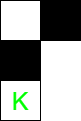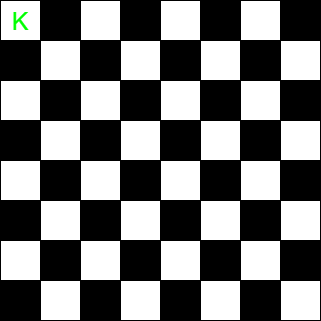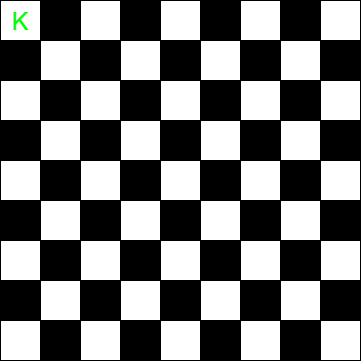# Puzzle | Can a Knight reach bottom from top by visiting all squares

• Difficulty Level : Medium
• Last Updated : 12 Oct, 2020

Puzzle
Is there a way for a chess knight to start at the top-left corner from a N x N chessboard, visit all the squares of the board exactly once and end at the lower-right corner.

Solution

We need to traverse all the squares of the chessboard exactly once. Also, the moves of the knight are L-shaped, that is, traverse two squares vertically or horizontally. A simple observation to take from this is that in one move, the knight starts and ends at two different colored squares.Consider two cases:

1. Case N is even: Let the value of N be 8. Hence, total number of squares on the board will be 64. Now, to visit all the squares of the board exactly once the knight would have to make 63 moves. Since, total number of moves are odd, the journey will start and end on the squares of the opposite color. Since, the squares on the top-left corner and lower-right corner are both coloured the same, hence the journey is impossible.2. Case N is odd: Let the value of N be 9. Hence, total number of squares on the board will be 81. Now, to visit all the squares of the board exactly once the knight would have to make 80 moves. Since, total number of moves are even, the journey will start and end on the squares of the same color. Since, the squares on the upper-left corner and lower-right corner are of the same color, hence the journey on 9 x 9 board is possible. But for N = 3, this is no solution as the knight cannot reach (2,2) position (which is the middle of chessboard).Hence, on a N x N chessboard, a knight will have to make (N2 – 1) moves to visit each square exactly once. So, if N is even, the journey is impossible and for odd values of N, the journey is possible.

My Personal Notes arrow_drop_up